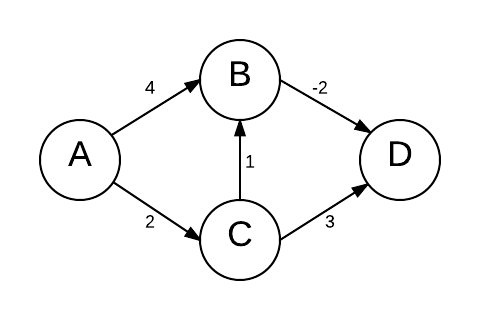# What will be the shortest path from $A$ to $D$ after reweighting?

Look at the following graph. Which path will be the shortest path from $A$ to $D$ after reweighting it with the technique used by Johnson's Algorithm?Graph with 4 vertices

×